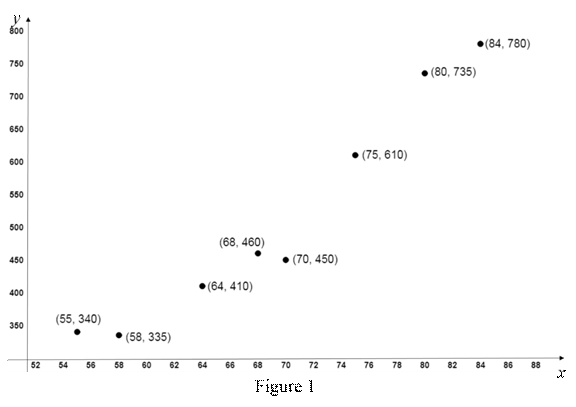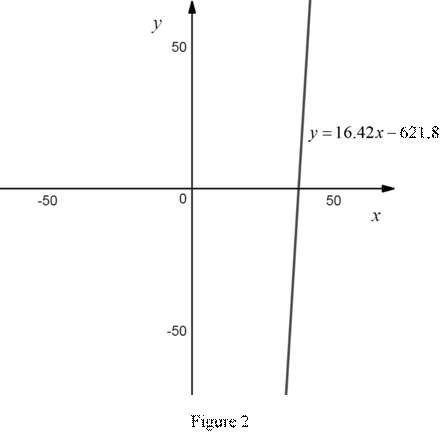# To graph: The scatter plot of the data.### Precalculus: Mathematics for Calcu...

6th Edition
Stewart + 5 others
Publisher: Cengage Learning
ISBN: 9780840068071### Precalculus: Mathematics for Calcu...

6th Edition
Stewart + 5 others
Publisher: Cengage Learning
ISBN: 9780840068071

#### Solutions

Chapter 1, Problem 2P

(a)

To determine

## To graph: The scatter plot of the data.

Expert Solution

### Explanation of Solution

Graph:

Consider the High temperature as the x coordinates and number of cans sold as the y coordinates.

The scatter plots of the given data are shown below in Figure 1.From Figure 1, all the points are plotted on the graph. No two points coincide each other.

(b)

To determine

### To find: The linear function that represents the given data and draw the graph for it.

Expert Solution

The linear function that represents the given data is y=16.42x621.8 .

### Explanation of Solution

Using the online calculator, the regression line for the given data obtained is y=16.42x621.8 , where y is the temperature and x is the number of cans sold.

The above regression line is in the form of linear equation.

Therefore, the linear function that represents the given data is y=16.42x621.8 .

The graph that represents the equation y=16.42x621.8 is shown below in Figure 2.From Figure 2, the graph for the linear equation y=16.42x621.8 is a straight line.

(c)

To determine

### To find: The number of soft drink sold if the temperature is 95°F .

Expert Solution

The number of soft drink sold if the temperature is 95°F is 938.

### Explanation of Solution

Consider the temperature is 95°F .

From part (b), it is obtained that the linear equation that represents the given data is y=16.42x621.8 .

Substitute x = 95 in the above equation to compute the number of cans sold.

y=16.42(95)621.8=1559.9621.8=938.1

Thus, the number of soft drink sold if the temperature is 95°F is 938.

### Have a homework question?

Subscribe to bartleby learn! Ask subject matter experts 30 homework questions each month. Plus, you’ll have access to millions of step-by-step textbook answers!